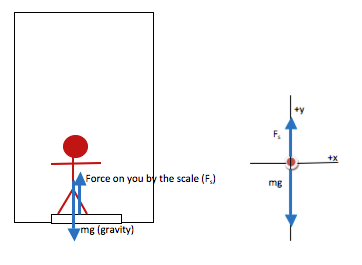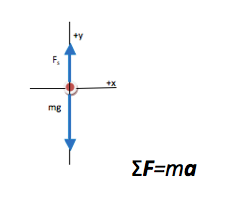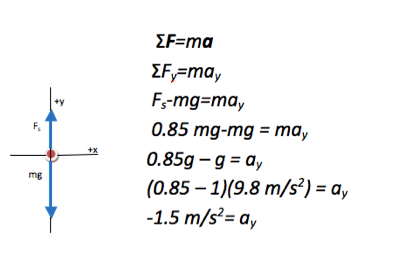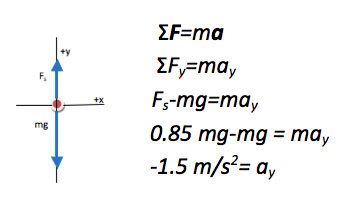# University of Wisconsin Green Bay

You are standing on a scale in an elevator on the 4th floor of the science building. As the elevator begins to descend to the first floor, you notice that the scale reads only 85% of your weight. What is the acceleration of the elevator during that period of time?

• In this problem, you are asked to relate motion (the acceleration of the elevator and the objects in it) to force (your weight and the contact between you and the scale). Force and motion of a single object are always related through Newton’s Second Law, so this is a force or 2nd Law problem.

••The key equation for any problem that relates forces and motion is Newton's Second Law. Regardless of what quantity you are asked to find, begin with the Second Law. If additional information is needed, it will become apparent as you proceed.

•Acceleration (your acceleration is the same as the elevator’s since you move together) is the quantity that was requested in the problem and so no further mathematical steps are needed. Note that the problem would have been worked in exactly the same way if acceleration was given and the reading of the scale was requested.

•You know from experience that when an elevator first begins to move downward, you feel lighter. This is because during that initial motion, the elevator has an acceleration in the downward direction. (Note that acceleration in your solution has a negative sign, which reflects the fact that the direction is down.) This downward acceleration means the floor (or scale, in this case) does not press into you as hard because it does not fully support you against gravity. That is reflected in this problem by the fact that the force on you by the scale is only 85% or your weight.

If you were in free fall, your acceleration would be -9.8 m/s. In this case, your actual acceleration is much less than that, and so the answer appears reasonable.